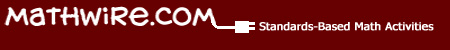Links to Math Topics on the Internet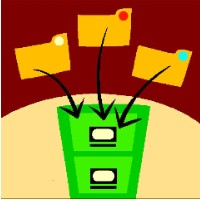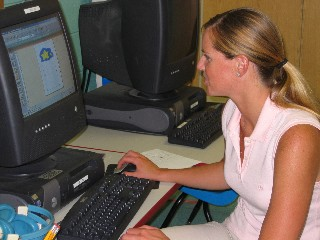## Mathwire.com Topics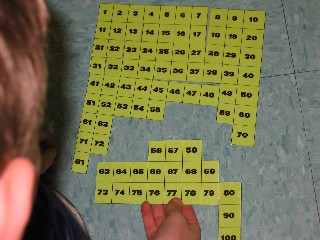## Number & Operations

These activities support student development of number sense and the concept of mathematical operations: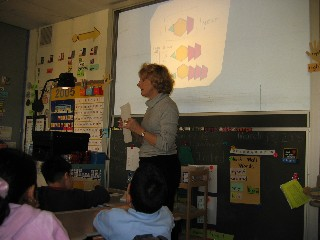## Algebra

These activities support student development of the concept of patterns through concrete activities with manipulatives,   The use of variables is developed as a mathematical way of recording the general rule.## Geometry

These activities support student development of spatial sense and foster familiarity with the mathematical vocabulary of geometry.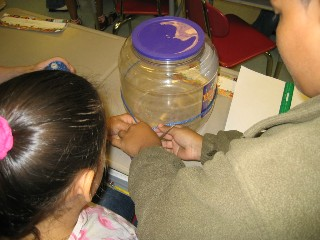## Measurement

These activities help students make sense of measurement by measuring the world around them using appropriate units. The emphasis is on constructing meaning for the units as well as the instruments students use to measure length, capacity, etc.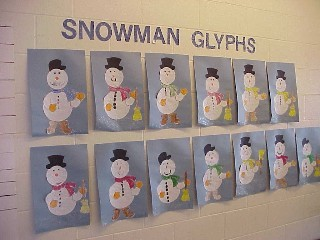## Data Analysis & Probability

These activities support students as they answer questions about the world around them. Learning to organize and analyze data are important life skills that students will use in both their professional and personal lives.## Discrete Math

These activities help students develop an appreciation of how discrete math is used to represent and solve problems in real-life situations.

## Problem Solving

These open-ended problem solving tasks challenge students to apply mathematical concepts and language to everyday problems.   Students also learn to explain their thinking using words, pictures and numbers.   The use of a problem-solving rubric helps students target a best performance by using the prompts found in the rubric.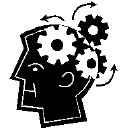These links challenge students to develop logical thinking and use patterns to solve these puzzles and brain teasers.